# NCERT Solutions for Class 12 Maths Chapter 13 Probability Ex 13.2

## NCERT Solutions for Class 12 Maths Chapter 13 Probability Ex 13.2

The topics and sub-topics included in Chapter 13 Probability the following:

 Section Name Topic Name 13 Probability 13.1 Introduction 13.2 Conditional Probability 13.3 Multiplication Theorem on Probability 13.4 Independent Events 13.5 Bayes’ Theorem 13.6 Random Variables and its Probability Distributions 13.7 Bernoulli Trials and Binomial Distribution

NCERT Solutions for Class 12 Maths Chapter 13 Probability 13.2 are part of NCERT Solutions for Class 12 Maths. Here we have given Class 12 Maths NCERT Solutions Probability Ex 13.2

Question 1
If, P(A) =3/5 and P(B) =1/5 find P (A ∩ B) if A and B are independent events.
Solution: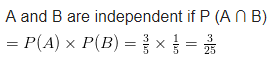Question 2.
Two cards are drawn at random and without replacement from a pack of 52 playing cards.Find the probability that both the cards are black.
Solution: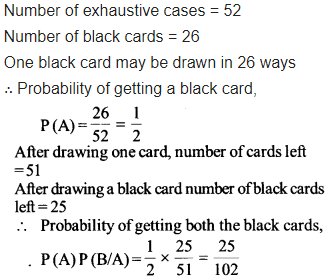Question 3.
A box of oranges is inspected by examining three randomly selected oranges drawn without replacement. If all the three oranges are good, the box is approved for sale otherwise it is rejected. Find the probability that a box containing 15 oranges out of which 12 are good and 3 are bad ones will be approved for sale.
Solution: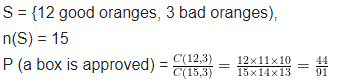Question 4.
A fair coin and an unbiased die are tossed. Let A be the event ‘head appears on the coin’ and B be the event ‘3 on the die’. Check whether A and B are independent events or not
Solution: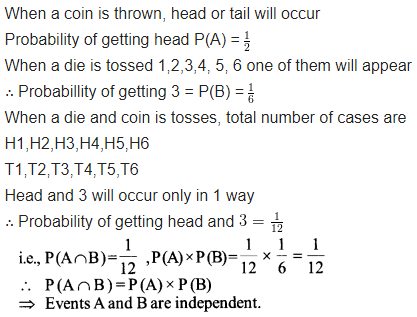Question 5.
A die marked 1,2,3 in red and 4,5,6 in green is tossed. Let A be the event, ‘the number is even’, and B be the event, ‘the number is red’. Are A and B independent?
Solution:Question 6.
Let E and F be the events with P(E) =$\frac { 3 }{ 5 }$, P (F) =$\frac { 3 }{ 10 }$and P (E ∩ F) =$\frac { 1 }{ 5 }$. Are E and F independent?
Solution: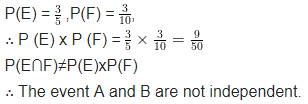Question 7.
Given that the events A and B are such that P(A) =$\frac { 1 }{ 2 }$,P(A∪B) =$\frac { 3 }{ 5 }$and P(B) = p. Find p if they are
(i) mutually exclusive
(ii) independent.
Sol: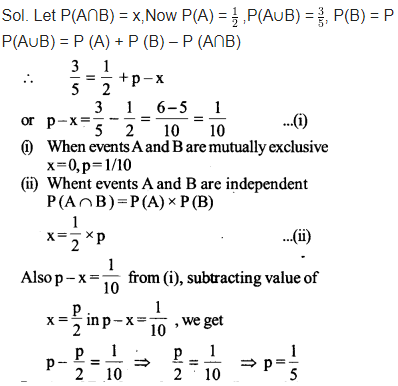Question 8.
Let A and B independent events P(A) = 0.3 and P(B) = 0.4. Find
(i) P(A∩B)
(ii) P(A∪B)
(iii) P (A | B)
(iv) P(B | A)
Solution: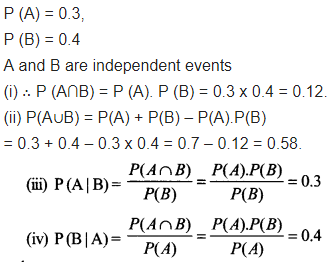Question 9.
If A and B are two events, such that P (A) =$\frac { 1 }{ 4 }$, P(B) =$\frac { 1 }{ 2 }$,and P(A∩B) =$\frac { 1 }{ 8 }$.Find P (not A and not B)
Solution: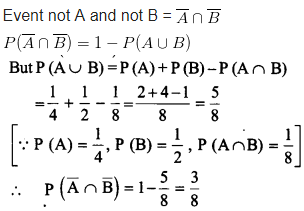Question 10
Events A and B are such that
P(A) =$\frac { 1 }{ 2 }$,P(B) =$\frac { 7 }{ 12 }$and P (not A or not B) =$\frac { 1 }{ 4 }$. State whether A and Bare independent
Solution:Question 11
Given two independent events A and B such that P (A) = 0.3, P(B) = 0.6. Find
(i) P(A and B)
(ii) P(A and not B)
(iii) P (A or B)
(iv) P (neither A nor B)
Solution: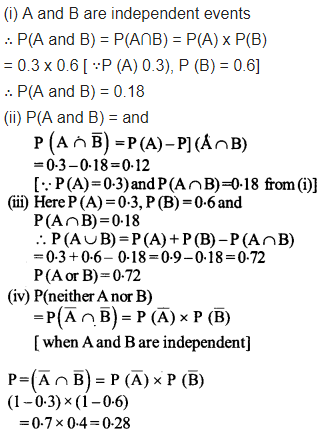Question 12
A die is tossed thrice. Find the probability of getting an odd number at least once.
Solution: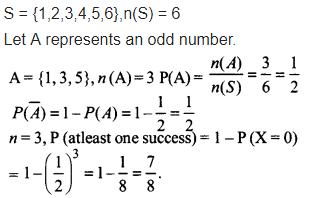Question 13
Two balls are drawn at random with replacement from a box containing 10 black and 8 red balls. Find the probability that
(i) both balls are red.
(ii) the first ball is “black and the second is red.
(iii) one of them is black and other is red.
Solution: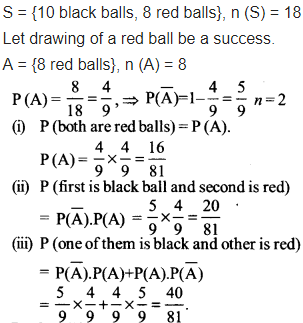Question 14
Probability of solving specific problem independently by A and B are$\frac { 1 }{ 2 }$and$\frac { 1 }{ 3 }$respectively. If both try to solve the problem independently, find the probability that
(i) the problem is solved
(ii) exactly one of them solves the problem.
Solution: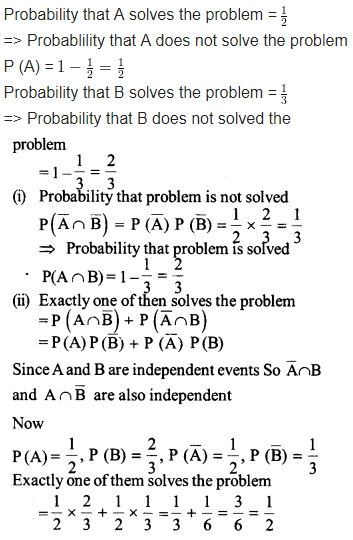Question 15
One card is drawn at random from a well-shuffled deck of 52 cards. In which of the following cases are the events E and F independent?
(i) E: ‘the card drawn is a spade’
F: ‘the card drawn is an ace ’
(ii) E: ‘the card drawn is black’
F: ‘the card drawn is a king’
(iii) E: ‘the card drawn is a king or queen ’
F: ‘the card drawn is a queen or jack ’.
Solution: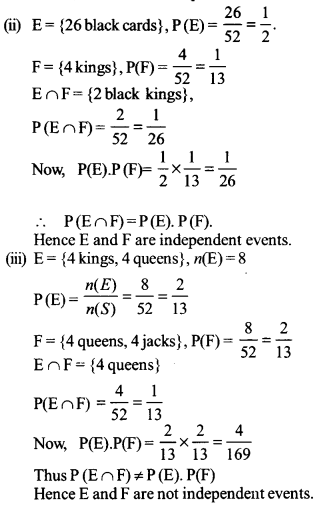Question 16
In a hostel, 60% of the students read Hindi newspaper, 40% read English newspaper and 20% read both Hindi and English newspapers. A student is selected at random
(a) Find the probability that she reads neither Hindi nor English newspapers.
(b) If she reads Hindi newspaper, find the probability that she reads english newspapers.
(c) If she reads English newspaper, find the probability that she reads Hindi newspaper.
Solution: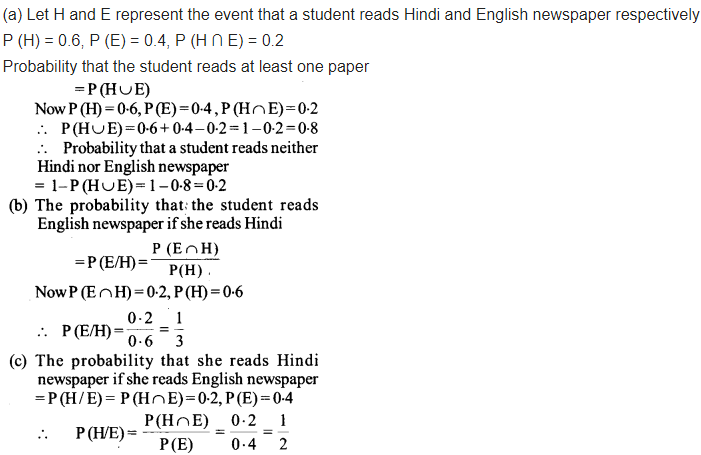Choose the correct answer in the following Question 17 and 18:

Question 17
The probability of obtaining an even prime number on each die when a pair of dice is rolled is
(a) 0
(b)$\frac { 1 }{ 3 }$
(c)$\frac { 1 }{ 12 }$
(d)$\frac { 1 }{ 36 }$
Solution:Question 18
Two events A and B are said to be independent, if
(a) A and B are mutually exclusive
(b) P(A’B’) = [1 – P(A)] [1 – P(B)]
(c) P(A) = P(B)
(d) P (A) + P (B) = 1
Solution: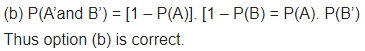+